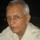Coffee Room
Discuss anything here - everything that you wish to discuss with fellow engineers.
12921 Members
Join this group to post and comment.zaveri • Mar 12, 2012

# How Is Brake Force Determined?

hi all

how is the braking force of a brake system of an automobile determined and how is the distance travelled after braking calculated. what is the data required besides the mass of the automobile and the deccelerationCE Designer • Mar 12, 2012
zaveri
hi all

how is the distance travelled after braking calculated. what is the data required besides the mass of the automobile and the decceleration
I am assuming you mean what is the distance travelled after coming to rest.
From particle motion i can see that you will need:
1. Velocity at the instant the brake was applied
Simply apply the formula v^2 = u^2 - 2(a)(s)
where v = 0

I am not certain how the mass of the car would come in here, maybe someone can help us.